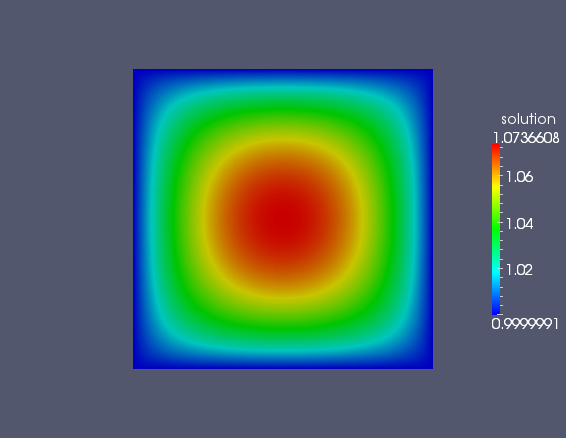# Post-processing Results with igakit¶

Objective: At the end of this tutorial you will understand how to output solution vectors from PetIGA and create VTK plots using igakit

Assumptions: I assume that in addition to PetIGA and PETSc, you have igakit installed. You should be comfortable with commandline options. Also, I assume some familiarity with python.

## Poisson Problem¶

To begin this tutorial, compile the demo code `\$PETIGA_DIR/demo/Poisson.c` and then run with these options:

```./Poisson -draw -draw_pause 3
```

This will execute the Poission solver and give you a rough plot of the solution for 3 seconds. The problem setup is such that we have unit Dirichlet boundary conditions on all boundaries and a unit forcing. You should be able to see this in the plot which is drawn. Here we are using a built-in viewer of PETSc to plot the control points of the solution using:

```VecView(x,PETSC_VIEWER_DRAW_WORLD);
```

where x was our solution vector. This is fine for visual confirmation of correctness in the eyeball norm, but for publications or talks we typically desire higher resolution graphics. For this, we need to output the discretization information and the solution vector and then post-process this. Rerun the example:

```./Poisson -save
```

Running with this option will additionally execute two lines in the code:

```IGAWrite(iga,"PoissonGeometry.dat");
IGAWriteVec(iga,x,"PoissonSolution.dat");
```

These lines dump the needed information into these datafiles.

## Python Script¶

Now, locate the file `\$PETIGA_DIR/demo/PoissonPlot.py` and examine its contents. We are using a few features of the python package igakit to read in and parse the discretization information. First we import the functionality we need:

```from igakit.io import PetIGA,VTK
from numpy import linspace
```

Igakit has a input/ouput module and so we grab the PetIGA and VTK functions from this module. We will also grab a Matlab-like linspace command from numpy. Then we need to read in our data files:

```nrb = PetIGA().read("PoissonGeometry.dat")
```

The first of these reads in the discretization information and geometry. If no geometry was used, then the control points will be the Greville abscissa. This gets stored as an igakit NURBS object into the variable `nrb`. There is a lot you can do with this object, but for now we will focus only on writing VTK files. The second line reads in the solution vector, but requires the NURBS object as well. This is so we know how to reshape the linear vector array into something that matches the discretization. Then we can write the VTK file

```VTK().write("PoissonVTK.vtk",       # output filename
nrb,                    # igakit NURBS object
fields=sol,             # sol is the numpy array to plot
sampler=uniform,        # specify the function to sample points
scalars={'solution':0}) # adds a scalar plot to the VTK file
```

By specifying `fields=sol` here, we are plotting the solution vector on top of the geometry contained in the `nrb` variable. Associating the solution vector to the plot is not enough to get the solution plotted in the VTK file. Here, we also specify:

```scalars={'solution':0}
```

where the target of this assignment is a dictionary. Basically a scalar plot will be created per entry in the dictionary. The string portion will be what the variable is called in the VTK plot and the integer portion indicates which component to use of the `sol` vector. In this case, we are solving a scalar problem and so there is only one choice.

The sampler line of this VTK call is not needed. By default, a VTK file will be written, interpolating the solution and geometry at the boundaries of non-zero knot spans. We call these breaks in igakit. However, we can specify a function here to create a finer resolution plot as well. We coded a funtion:

```uniform = lambda U: linspace(U, U[-1], 100)
```

where `U` will be the breaks in the B-spline space in each dimension. This function simply returns 100 points in between the first and the last break. This will create a finely interpolated plot. By executing this python script:

```python PoissonPlot.py
```

you will generate a VTK file called `PoissonVTK.vtk`. This you can view with many free viewers such as Paraview or VisIt. Your plot should look something like the following figure.## Vector Problems¶

If your problems are vector-valued, then you can output the components as vector objects in VTK. Compile and execute the `\$PETIGA_DIR/demo/NavierStokesKorteweg2D.c` code:

```make NavierStokesKorteweg2D
./NavierStokesKorteweg2D -nsk_output -ts_max_steps 0
```

This will run the code with the output option on for zero time steps. We will get a vector out of this code which corresponds to the initial condition. However, this problem has three variables: the first is the density and the last two are the components of the velocity. We can plot these by sightly adjusting our python script:

```from igakit.io import PetIGA,VTK
from numpy import linspace
uniform = lambda U: linspace(U, U[-1], 100)
VTK().write("nsk0.vtk",
nrb,
fields=sol,
sampler=uniform,
scalars={'density':0},
vectors={'velocity':[1,2]})
```

The main difference is that now we have added a `vectors` line along with a list of indices. Intuitively, these components align with their location in the `sol` vector as before. Now the resulting VTK file will contain multiple components for plotting.

## Time Dependent Problems¶

If you let the NavierStokesKorteweg code run for more steps (say `-ts_max_steps 10`) then you will get more datafiles of the form `nsk2d*.dat`. We can slightly edit our script then to convert all time steps using the glob module:

```from igakit.io import PetIGA,VTK
from numpy import linspace
import glob
The main difference is that we create a list of files to process using glob and read the solution vector inside of this loop. The output filename is then created from the input by removing the extension and adding `.vtk`.